# Convert Infix expression to Postfix expression

Write a program to convert an Infix expression to Postfix form.

Infix expression: The expression of the form “a operator b” (a + b) i.e., when an operator is in-between every pair of operands.
Postfix expression: The expression of the form “a b operator” (ab+) i.e., When every pair of operands is followed by an operator.

Examples:

Input: A + B * C + D
Output: ABC*+D+

Input: ((A + B) – C * (D / E)) + F
Output: AB+CDE/*-F+

## Why postfix representation of the expression?

The compiler scans the expression either from left to right or from right to left.
Consider the expression: a + b * c + d

• The compiler first scans the expression to evaluate the expression b * c, then again scans the expression to add a to it.
• The result is then added to d after another scan.

The repeated scanning makes it very inefficient. Infix expressions are easily readable and solvable by humans whereas the computer cannot differentiate the operators and parenthesis easily so, it is better to convert the expression to postfix(or prefix) form before evaluation.

The corresponding expression in postfix form is abc*+d+. The postfix expressions can be evaluated easily using a stack.

## How to convert an Infix expression to a Postfix expression?

To convert infix expression to postfix expression, use the stack data structure. Scan the infix expression from left to right. Whenever we get an operand, add it to the postfix expression and if we get an operator or parenthesis add it to the stack by maintaining their precedence.

Below are the steps to implement the above idea:

1. Scan the infix expression from left to right
2. If the scanned character is an operand, put it in the postfix expression.
3. Otherwise, do the following
• If the precedence and associativity of the scanned operator are greater than the precedence and associativity of the operator in the stack [or the stack is empty or the stack contains a ‘(‘ ], then push it in the stack. [‘^‘ operator is right associative and other operators like ‘+‘,’‘,’*‘ and ‘/‘ are left-associative].
• Check especially for a condition when the operator at the top of the stack and the scanned operator both are ‘^‘. In this condition, the precedence of the scanned operator is higher due to its right associativity. So it will be pushed into the operator stack.
• In all the other cases when the top of the operator stack is the same as the scanned operator, then pop the operator from the stack because of left associativity due to which the scanned operator has less precedence.
• Else, Pop all the operators from the stack which are greater than or equal to in precedence than that of the scanned operator.
• After doing that Push the scanned operator to the stack. (If you encounter parenthesis while popping then stop there and push the scanned operator in the stack.)
4. If the scanned character is a ‘(‘, push it to the stack.
5. If the scanned character is a ‘)’, pop the stack and output it until a ‘(‘ is encountered, and discard both the parenthesis.
6. Repeat steps 2-5 until the infix expression is scanned.
7. Once the scanning is over, Pop the stack and add the operators in the postfix expression until it is not empty.
8. Finally, print the postfix expression.

### Illustration:

Follow the below illustration for a better understanding

Consider the infix expression exp = “a+b*c+d”
and the infix expression is scanned using the iterator i, which is initialized as i = 0.

1st Step: Here i = 0 and exp[i] = ‘a’ i.e., an operand. So add this in the postfix expression. Therefore, postfix = “a”.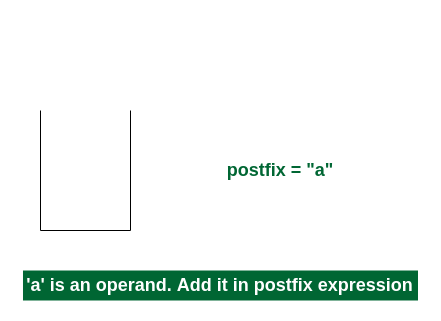2nd Step: Here i = 1 and exp[i] = ‘+’ i.e., an operator. Push this into the stack. postfix = “a” and stack = {+}.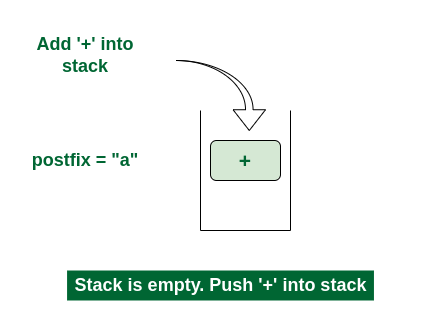Push ‘+’ in the stack

3rd Step: Now i = 2 and exp[i] = ‘b’ i.e., an operand. So add this in the postfix expression. postfix = “ab” and stack = {+}.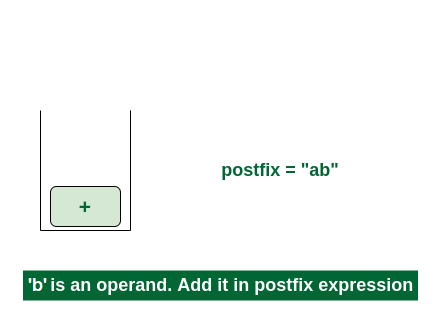4th Step: Now i = 3 and exp[i] = ‘*’ i.e., an operator. Push this into the stack. postfix = “ab” and stack = {+, *}.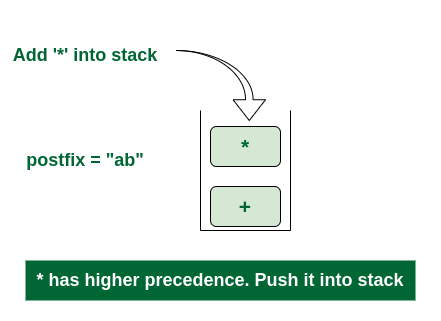Push ‘*’ in the stack

5th Step: Now i = 4 and exp[i] = ‘c’ i.e., an operand. Add this in the postfix expression. postfix = “abc” and stack = {+, *}.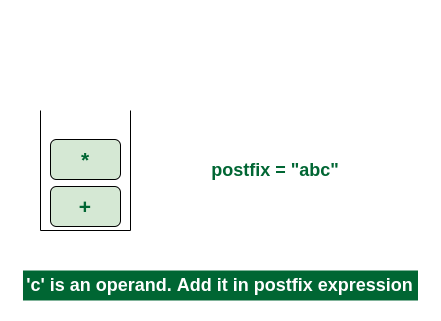6th Step: Now i = 5 and exp[i] = ‘+’ i.e., an operator. The topmost element of the stack has higher precedence. So pop until the stack becomes empty or the top element has less precedence. ‘*’ is popped and added in postfix. So postfix = “abc*” and stack = {+}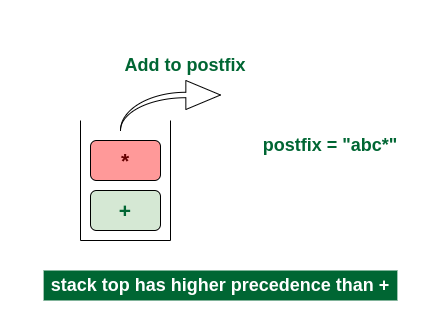Pop ‘*’ and add in postfix

Now top element is ‘+‘ that also doesn’t have less precedence. Pop it. postfix = “abc*+”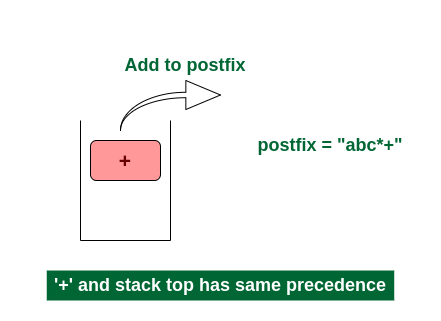Pop ‘+’ and add it in postfix

Now stack is empty. So push ‘+’ in the stack. stack = {+}.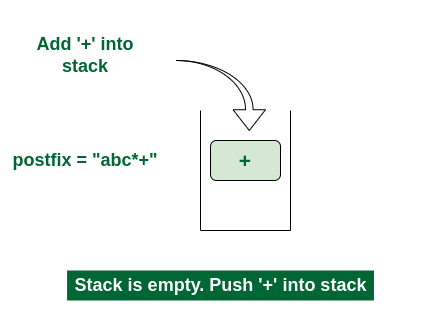Push ‘+’ in the stack

7th Step: Now i = 6 and exp[i] = ‘d’ i.e., an operand. Add this in the postfix expression. postfix = “abc*+d”.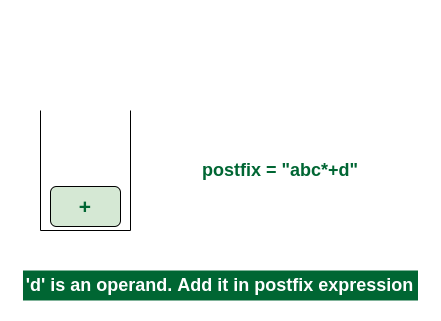Final Step: Now no element is left. So empty the stack and add it in the postfix expression. postfix = “abc*+d+”.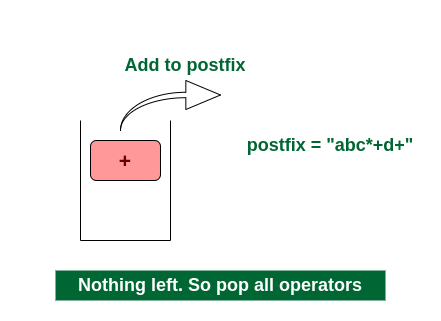Pop ‘+’ and add it in postfix

Below is the implementation of the above algorithm:

## C++14

 `// C++ code to convert infix expression to postfix`   `#include ` `using` `namespace` `std;`   `// Function to return precedence of operators` `int` `prec(``char` `c)` `{` `    ``if` `(c == ``'^'``)` `        ``return` `3;` `    ``else` `if` `(c == ``'/'` `|| c == ``'*'``)` `        ``return` `2;` `    ``else` `if` `(c == ``'+'` `|| c == ``'-'``)` `        ``return` `1;` `    ``else` `        ``return` `-1;` `}`   `// The main function to convert infix expression` `// to postfix expression` `void` `infixToPostfix(string s)` `{`   `    ``stack<``char``> st;` `    ``string result;`   `    ``for` `(``int` `i = 0; i < s.length(); i++) {` `        ``char` `c = s[i];`   `        ``// If the scanned character is` `        ``// an operand, add it to output string.` `        ``if` `((c >= ``'a'` `&& c <= ``'z'``) || (c >= ``'A'` `&& c <= ``'Z'``)` `            ``|| (c >= ``'0'` `&& c <= ``'9'``))` `            ``result += c;`   `        ``// If the scanned character is an` `        ``// ‘(‘, push it to the stack.` `        ``else` `if` `(c == ``'('``)` `            ``st.push(``'('``);`   `        ``// If the scanned character is an ‘)’,` `        ``// pop and add to output string from the stack` `        ``// until an ‘(‘ is encountered.` `        ``else` `if` `(c == ``')'``) {` `            ``while` `(st.top() != ``'('``) {` `                ``result += st.top();` `                ``st.pop();` `            ``}` `            ``st.pop();` `        ``}`   `        ``// If an operator is scanned` `        ``else` `{` `            ``while` `(!st.empty()` `                   ``&& prec(s[i]) <= prec(st.top())) {` `                ``result += st.top();` `                ``st.pop();` `            ``}` `            ``st.push(c);` `        ``}` `    ``}`   `    ``// Pop all the remaining elements from the stack` `    ``while` `(!st.empty()) {` `        ``result += st.top();` `        ``st.pop();` `    ``}`   `    ``cout << result << endl;` `}`   `// Driver code` `int` `main()` `{` `    ``string ``exp` `= ``"a+b*(c^d-e)^(f+g*h)-i"``;`   `    ``// Function call` `    ``infixToPostfix(``exp``);` `  `  `    ``return` `0;` `}`

## C

 `// C code to convert infix to postfix expression`   `#include ` `#include ` `#include `   `#define MAX_EXPR_SIZE 100`   `// Function to return precedence of operators` `int` `precedence(``char` `operator)` `{` `    ``switch` `(operator) {` `    ``case` `'+'``:` `    ``case` `'-'``:` `        ``return` `1;` `    ``case` `'*'``:` `    ``case` `'/'``:` `        ``return` `2;` `    ``case` `'^'``:` `        ``return` `3;` `    ``default``:` `        ``return` `-1;` `    ``}` `}`   `// Function to check if the scanned character ` `// is an operator` `int` `isOperator(``char` `ch)` `{` `    ``return` `(ch == ``'+'` `|| ch == ``'-'` `|| ch == ``'*'` `|| ch == ``'/'` `            ``|| ch == ``'^'``);` `}`   `// Main functio to convert infix expression` `// to postfix expression` `char``* infixToPostfix(``char``* infix)` `{` `    ``int` `i, j;` `    ``int` `len = ``strlen``(infix);` `    ``char``* postfix = (``char``*)``malloc``(``sizeof``(``char``) * (len + 2));` `    ``char` `stack[MAX_EXPR_SIZE];` `    ``int` `top = -1;`   `    ``for` `(i = 0, j = 0; i < len; i++) {` `        ``if` `(infix[i] == ``' '` `|| infix[i] == ``'\t'``)` `            ``continue``;` `      `  `        ``// If the scanned character is operand` `        ``// add it to the postfix expression` `        ``if` `(``isalnum``(infix[i])) {` `            ``postfix[j++] = infix[i];` `        ``}` `      `  `        ``// if the scanned character is '('` `        ``// push it in the stack` `        ``else` `if` `(infix[i] == ``'('``) {` `            ``stack[++top] = infix[i];` `        ``}` `      `  `        ``// if the scanned character is ')'` `        ``// pop the stack and add it to the ` `        ``// output string until empty or '(' found` `        ``else` `if` `(infix[i] == ``')'``) {` `            ``while` `(top > -1 && stack[top] != ``'('``)` `                ``postfix[j++] = stack[top--];` `           `  `                ``top--;` `        ``}` `      `  `        ``// If the scanned character is an operator` `        ``// push it in the stack` `        ``else` `if` `(isOperator(infix[i])) {` `            ``while` `(top > -1` `                   ``&& precedence(stack[top])` `                          ``>= precedence(infix[i]))` `                ``postfix[j++] = stack[top--];` `            ``stack[++top] = infix[i];` `        ``}` `    ``}`   `    ``// Pop all remaining elements from the stack` `    ``while` `(top > -1) {` `        ``if` `(stack[top] == ``'('``) {` `            ``return` `"Invalid Expression"``;` `        ``}` `        ``postfix[j++] = stack[top--];` `    ``}` `    ``postfix[j] = ``'\0'``;` `    ``return` `postfix;` `}`   `// Driver code` `int` `main()` `{` `    ``char` `infix[MAX_EXPR_SIZE] = ``"a+b*(c^d-e)^(f+g*h)-i"``;` `  `  `    ``// Function call` `    ``char``* postfix = infixToPostfix(infix);` `    ``printf``(``"%s\n"``, postfix);` `    ``free``(postfix);` `    ``return` `0;` `}`

## Java

 `// Java code to convert infix expression to postfix`   `import` `java.util.ArrayDeque;` `import` `java.util.Deque;` `import` `java.util.Stack;`   `class` `Test {`   `    ``// A utility function to return` `    ``// precedence of a given operator` `    ``// Higher returned value means` `    ``// higher precedence` `    ``static` `int` `Prec(``char` `ch)` `    ``{` `        ``switch` `(ch) {` `        ``case` `'+'``:` `        ``case` `'-'``:` `            ``return` `1``;`   `        ``case` `'*'``:` `        ``case` `'/'``:` `            ``return` `2``;`   `        ``case` `'^'``:` `            ``return` `3``;` `        ``}` `        ``return` `-``1``;` `    ``}`   `    ``// The main method that converts` `    ``// given infix expression` `    ``// to postfix expression.` `    ``static` `String infixToPostfix(String exp)` `    ``{` `        ``// Initializing empty String for result` `        ``String result = ``new` `String(``""``);`   `        ``// Initializing empty stack` `        ``Deque stack` `            ``= ``new` `ArrayDeque();`   `        ``for` `(``int` `i = ``0``; i < exp.length(); ++i) {` `            ``char` `c = exp.charAt(i);`   `            ``// If the scanned character is an` `            ``// operand, add it to output.` `            ``if` `(Character.isLetterOrDigit(c))` `                ``result += c;`   `            ``// If the scanned character is an '(',` `            ``// push it to the stack.` `            ``else` `if` `(c == ``'('``)` `                ``stack.push(c);`   `            ``// If the scanned character is an ')',` `            ``// pop and output from the stack` `            ``// until an '(' is encountered.` `            ``else` `if` `(c == ``')'``) {` `                ``while` `(!stack.isEmpty()` `                       ``&& stack.peek() != ``'('``) {` `                    ``result += stack.peek();` `                    ``stack.pop();` `                ``}`   `                ``stack.pop();` `            ``}` `          `  `            ``// An operator is encountered` `            ``else` `            ``{` `                ``while` `(!stack.isEmpty()` `                       ``&& Prec(c) <= Prec(stack.peek())) {`   `                    ``result += stack.peek();` `                    ``stack.pop();` `                ``}` `                ``stack.push(c);` `            ``}` `        ``}`   `        ``// Pop all the operators from the stack` `        ``while` `(!stack.isEmpty()) {` `            ``if` `(stack.peek() == ``'('``)` `                ``return` `"Invalid Expression"``;` `            ``result += stack.peek();` `            ``stack.pop();` `        ``}` `      `  `        ``return` `result;` `    ``}`   `    ``// Driver code ` `    ``public` `static` `void` `main(String[] args)` `    ``{` `        ``String exp = ``"a+b*(c^d-e)^(f+g*h)-i"``;` `      `  `          ``// Function call` `        ``System.out.println(infixToPostfix(exp));` `    ``}` `}`

## Python3

 `# Python program to convert infix expression to postfix`     `# Class to convert the expression` `class` `Conversion:`   `    ``# Constructor to initialize the class variables` `    ``def` `__init__(``self``, capacity):` `        ``self``.top ``=` `-``1` `        ``self``.capacity ``=` `capacity` `        `  `        ``# This array is used a stack` `        ``self``.array ``=` `[]` `        `  `        ``# Precedence setting` `        ``self``.output ``=` `[]` `        ``self``.precedence ``=` `{``'+'``: ``1``, ``'-'``: ``1``, ``'*'``: ``2``, ``'/'``: ``2``, ``'^'``: ``3``}`   `    ``# Check if the stack is empty` `    ``def` `isEmpty(``self``):` `        ``return` `True` `if` `self``.top ``=``=` `-``1` `else` `False`   `    ``# Return the value of the top of the stack` `    ``def` `peek(``self``):` `        ``return` `self``.array[``-``1``]`   `    ``# Pop the element from the stack` `    ``def` `pop(``self``):` `        ``if` `not` `self``.isEmpty():` `            ``self``.top ``-``=` `1` `            ``return` `self``.array.pop()` `        ``else``:` `            ``return` `"\$"`   `    ``# Push the element to the stack` `    ``def` `push(``self``, op):` `        ``self``.top ``+``=` `1` `        ``self``.array.append(op)`   `    ``# A utility function to check is the given character` `    ``# is operand` `    ``def` `isOperand(``self``, ch):` `        ``return` `ch.isalpha()`   `    ``# Check if the precedence of operator is strictly` `    ``# less than top of stack or not` `    ``def` `notGreater(``self``, i):` `        ``try``:` `            ``a ``=` `self``.precedence[i]` `            ``b ``=` `self``.precedence[``self``.peek()]` `            ``return` `True` `if` `a <``=` `b ``else` `False` `        ``except` `KeyError:` `            ``return` `False`   `    ``# The main function that` `    ``# converts given infix expression` `    ``# to postfix expression` `    ``def` `infixToPostfix(``self``, exp):`   `        ``# Iterate over the expression for conversion` `        ``for` `i ``in` `exp:` `            `  `            ``# If the character is an operand,` `            ``# add it to output` `            ``if` `self``.isOperand(i):` `                ``self``.output.append(i)`   `            ``# If the character is an '(', push it to stack` `            ``elif` `i ``=``=` `'('``:` `                ``self``.push(i)`   `            ``# If the scanned character is an ')', pop and` `            ``# output from the stack until and '(' is found` `            ``elif` `i ``=``=` `')'``:` `                ``while``((``not` `self``.isEmpty()) ``and` `                      ``self``.peek() !``=` `'('``):` `                    ``a ``=` `self``.pop()` `                    ``self``.output.append(a)` `                ``if` `(``not` `self``.isEmpty() ``and` `self``.peek() !``=` `'('``):` `                    ``return` `-``1` `                ``else``:` `                    ``self``.pop()`   `            ``# An operator is encountered` `            ``else``:` `                ``while``(``not` `self``.isEmpty() ``and` `self``.notGreater(i)):` `                    ``self``.output.append(``self``.pop())` `                ``self``.push(i)`   `        ``# Pop all the operator from the stack` `        ``while` `not` `self``.isEmpty():` `            ``self``.output.append(``self``.pop())`   `        ``for` `ch ``in` `self``.output:` `            ``print``(ch, end``=``"")`     `# Driver code` `if` `__name__ ``=``=` `'__main__'``:` `    ``exp ``=` `"a+b*(c^d-e)^(f+g*h)-i"` `    ``obj ``=` `Conversion(``len``(exp))`   `    ``# Function call` `    ``obj.infixToPostfix(exp)`   `# This code is contributed by Nikhil Kumar Singh(nickzuck_007)`

## C#

 `// C# code to convert infix expression to postfix*/`   `using` `System;` `using` `System.Collections.Generic;`   `public` `class` `Test {`   `    ``// A utility function to return` `    ``// precedence of a given operator` `    ``// Higher returned value means higher precedence` `    ``internal` `static` `int` `Prec(``char` `ch)` `    ``{` `        ``switch` `(ch) {` `        ``case` `'+'``:` `        ``case` `'-'``:` `            ``return` `1;`   `        ``case` `'*'``:` `        ``case` `'/'``:` `            ``return` `2;`   `        ``case` `'^'``:` `            ``return` `3;` `        ``}` `        ``return` `-1;` `    ``}`   `    ``// The main method that converts given infix expression` `    ``// to postfix expression.` `    ``public` `static` `string` `infixToPostfix(``string` `exp)` `    ``{` `        ``// Initializing empty String for result` `        ``string` `result = ``""``;`   `        ``// Initializing empty stack` `        ``Stack<``char``> stack = ``new` `Stack<``char``>();`   `        ``for` `(``int` `i = 0; i < exp.Length; ++i) {` `            ``char` `c = exp[i];`   `            ``// If the scanned character is an` `            ``// operand, add it to output.` `            ``if` `(``char``.IsLetterOrDigit(c)) {` `                ``result += c;` `            ``}`   `            ``// If the scanned character is an '(',` `            ``// push it to the stack.` `            ``else` `if` `(c == ``'('``) {` `                ``stack.Push(c);` `            ``}`   `            ``// If the scanned character is an ')',` `            ``// pop and output from the stack` `            ``// until an '(' is encountered.` `            ``else` `if` `(c == ``')'``) {` `                ``while` `(stack.Count > 0` `                       ``&& stack.Peek() != ``'('``) {` `                    ``result += stack.Pop();` `                ``}`   `                ``if` `(stack.Count > 0` `                    ``&& stack.Peek() != ``'('``) {` `                    ``return` `"Invalid Expression"``;` `                ``}` `                ``else` `{` `                    ``stack.Pop();` `                ``}` `            ``}` `          `  `            ``// An operator is encountered` `            ``else` `            ``{` `                ``while` `(stack.Count > 0` `                       ``&& Prec(c) <= Prec(stack.Peek())) {` `                    ``result += stack.Pop();` `                ``}` `                ``stack.Push(c);` `            ``}` `        ``}`   `        ``// Pop all the operators from the stack` `        ``while` `(stack.Count > 0) {` `            ``result += stack.Pop();` `        ``}`   `        ``return` `result;` `    ``}`   `    ``// Driver code` `    ``public` `static` `void` `Main(``string``[] args)` `    ``{` `        ``string` `exp = ``"a+b*(c^d-e)^(f+g*h)-i"``;` `      `  `        ``// Function call` `        ``Console.WriteLine(infixToPostfix(exp));` `    ``}` `}`   `// This code is contributed by Shrikant13`

## Javascript

 `    ``/* Javascript implementation to convert` `    ``infix expression to postfix*/` `    `  `    ``//Function to return precedence of operators` `    ``function` `prec(c) {` `        ``if``(c == ``'^'``)` `            ``return` `3;` `        ``else` `if``(c == ``'/'` `|| c==``'*'``)` `            ``return` `2;` `        ``else` `if``(c == ``'+'` `|| c == ``'-'``)` `            ``return` `1;` `        ``else` `            ``return` `-1;` `    ``}`   `    ``// The main function to convert infix expression` `    ``//to postfix expression` `    ``function` `infixToPostfix(s) {`   `        ``let st = []; ``//For stack operations, we are using JavaScript built in stack` `        ``let result = ``""``;`   `        ``for``(let i = 0; i < s.length; i++) {` `            ``let c = s[i];`   `            ``// If the scanned character is` `            ``// an operand, add it to output string.` `            ``if``((c >= ``'a'` `&& c <= ``'z'``) || (c >= ``'A'` `&& c <= ``'Z'``) || (c >= ``'0'` `&& c <= ``'9'``))` `                ``result += c;`   `            ``// If the scanned character is an` `            ``// ‘(‘, push it to the stack.` `            ``else` `if``(c == ``'('``)` `                ``st.push(``'('``);`   `            ``// If the scanned character is an ‘)’,` `            ``// pop and to output string from the stack` `            ``// until an ‘(‘ is encountered.` `            ``else` `if``(c == ``')'``) {` `                ``while``(st[st.length - 1] != ``'('``)` `                ``{` `                    ``result += st[st.length - 1];` `                    ``st.pop();` `                ``}` `                ``st.pop();` `            ``}`   `            ``//If an operator is scanned` `            ``else` `{` `                ``while``(st.length != 0 && prec(s[i]) <= prec(st[st.length - 1])) {` `                    ``result += st[st.length - 1];` `                    ``st.pop(); ` `                ``}` `                ``st.push(c);` `            ``}` `        ``}`   `        ``// Pop all the remaining elements from the stack` `        ``while``(st.length != 0) {` `            ``result += st[st.length - 1];` `            ``st.pop();` `        ``}`   `        ``document.write(result + ``"
"``);` `    ``}` `    `  `    ``let exp = ``"a+b*(c^d-e)^(f+g*h)-i"``;` `    ``infixToPostfix(exp);`   `// This code is contributed by decode2207.`

Output

```abcd^e-fgh*+^*+i-

```

Time Complexity: O(N), where N is the size of the infix expression
Auxiliary Space: O(N), where N is the size of the infix expression